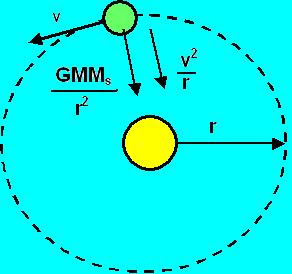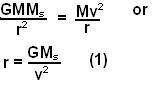## Wednesday, July 2, 2008

### Irodov Problem 1.200Let the mass of sun be Ms and the radius of orbit of the planet be r. The force of gravity acting on the planet is given by. This gravitational force provide for the centripetal acceleration of the planet and so we have,The total distance traveled by the planet in one rotation is the circumference of the circle and so the time taken for one rotation is given by,. Note that in Irodov the answer seems like the period of rotation depends on the mass of the planet M, but that false, it will depend on the mass of the central mass (sun in this case) and not on the mass of the planet.